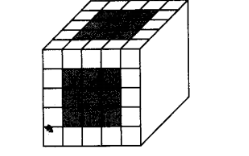# A cube of side 5 cm is painted on all its faces.

Question:

A cube of side 5 cm is painted on all its faces. If it is sliced into 1 cubic centimetre cubes, then how many 1 cubic centimetre cubes will

have exactly one of their faces painted?

(a) 27

(b) 42

(c) 54

(d) 142

Solution:

(c) Given, a cube of side $5 \mathrm{~cm}$ is painted on all its faces and is sliced into $1 \mathrm{~cm}^{3}$ cubes. Then, from figure, it is clear that

there are 9 cubes available on face.Since, there are six faces available.

Hence, total number of smaller cubes $=6 \times 9=54$